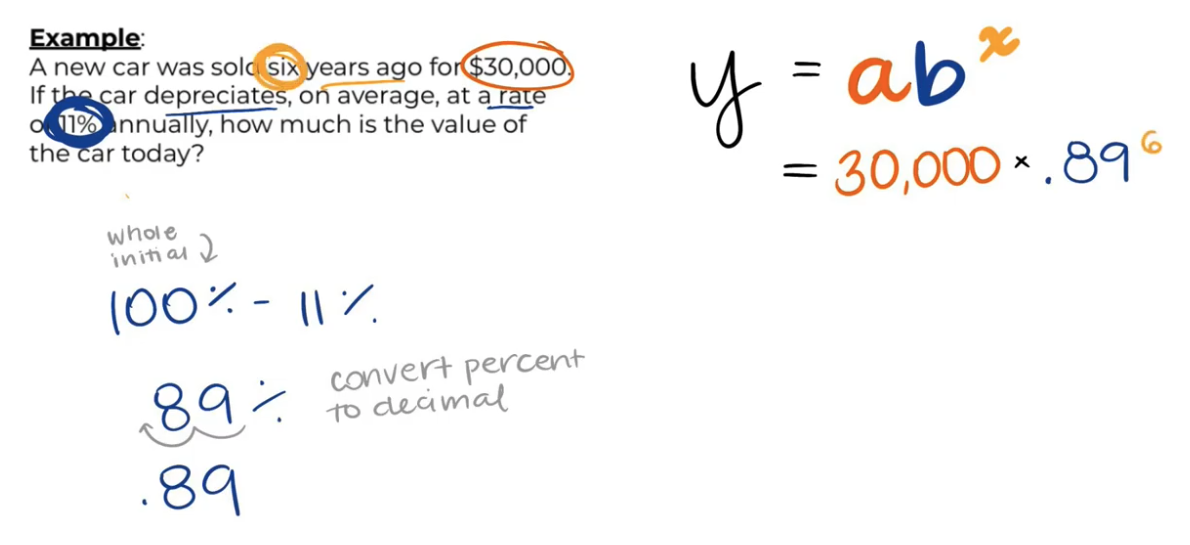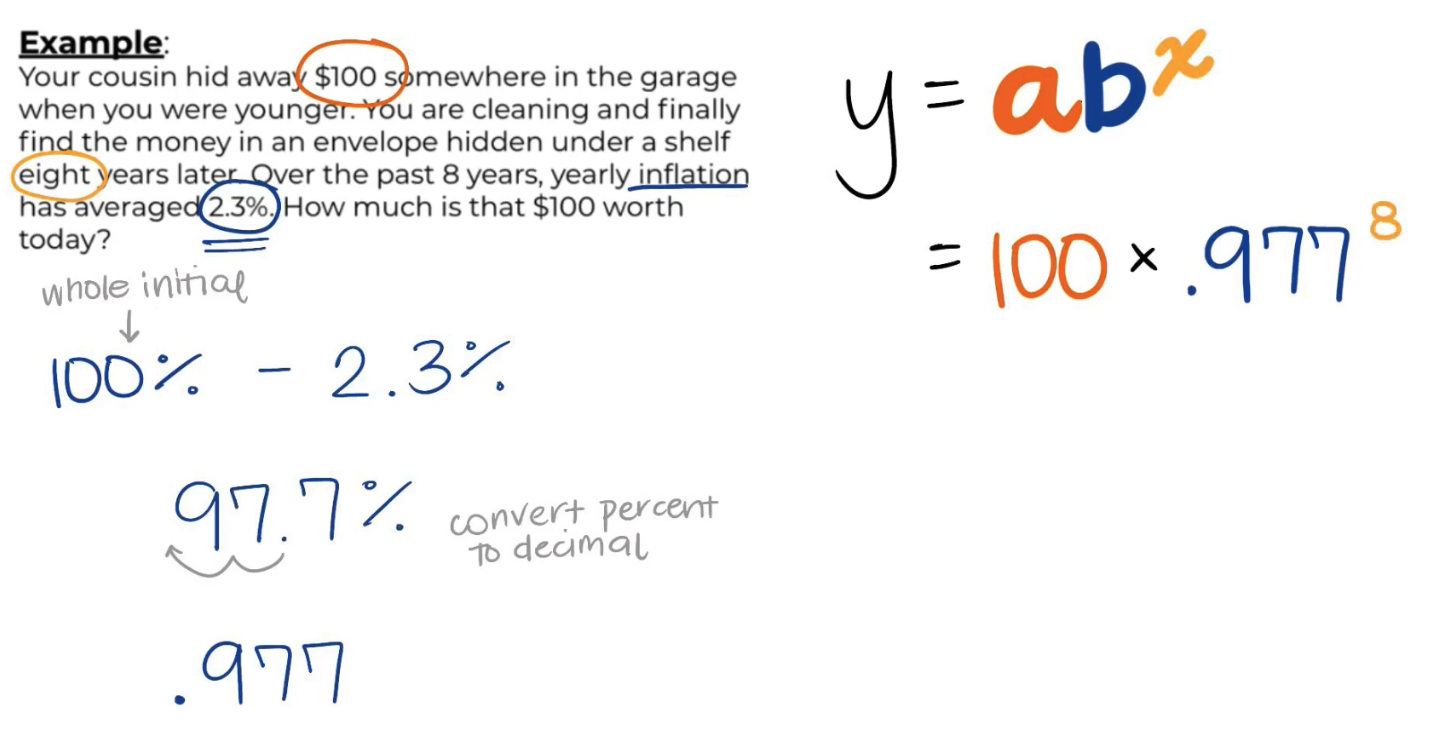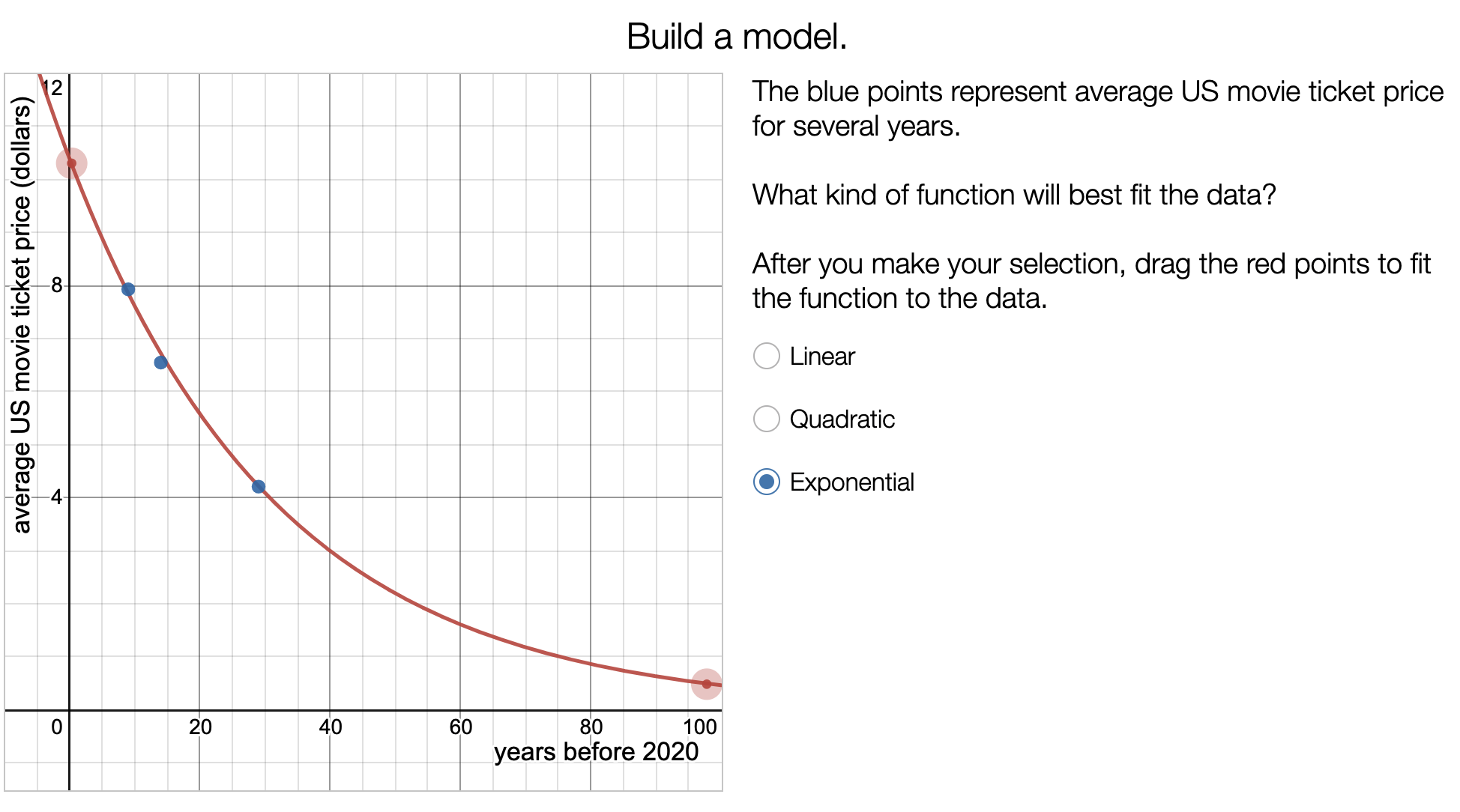May 16, 2021

### Math Monday: Two Real-World Applications of Exponential Decay

It can be hard to make exponential decay relevant for students - there are only so many times you can talk about the half-lives of radioactive isotopes. Today, we’ll walk through how you can make exponential functions engaging with cars, money, and candy.

Activity 1: Car Depreciation

In MATH: Depreciation of Car Value, students use the exponential decay formula to model how a car decreases in value due to depreciation over time.

Using the Edpuzzle video and example problem, students learn how to evaluate word problems with exponential decay, including calculating decay rate. Then, they practice similar word problems and create a graph of car value over time.In MATH: Exponential Decay in Purchasing Power, students learn how inflation impacts how much they can buy with their money. Start with the Edpuzzle video, which illustrates why stashing your money in the garage isn’t always a great idea. Students learn what inflation is, review exponential decay vs. growth, and calculate the present value of \$100 from 8 years ago.Then, students practice using the exponential decay equation. They consider the present value of uninvested cash, stagnant salaries, and periods of high inflation.

Building Engagement and Conceptual Understanding

Here are some fun ways you can supplement this activity to differentiate and deepen math learning.Extend the Personal Finance Learning

If you’re a math teacher, consider using these resources to learn more about personal finance and continue making real-world connections in math!

Sign up for Virtual PD to explore the NGPF's resources, brush up on content knowledge, and collaborate with other educators.